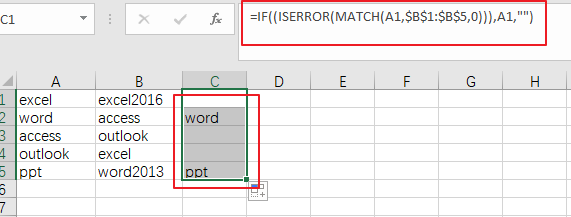# How to Compare Two Columns for Differences in Excel

This post will guide you how to compare two columns and return differences in Excel. How do I compare two columns to find differences in Excel 2013/2016.

## Compare Two Columns for Differences

Assuming that you have a list of data in range A1:B5, and you want to compare Column A and Column B and return the differences from these two columns. You can use an formula based on the IF function, the ISERROR function and the MATCH function to compare those two columns and find the differences.  like this:

if you want to get the differences between column A and Column B, just use the following formula:

=IF((ISERROR(MATCH(A1,\$B\$1:\$B\$5,0))),A1,””)

type this formula into a blank cell and press Enter key on your keyboard, and then drag the AutoFill Handle down to other cells.  You would see the differences.### Related Functions

• Excel IF function
The Excel IF function perform a logical test to return one value if the condition is TRUE and return another value if the condition is FALSE. The IF function is a build-in function in Microsoft Excel and it is categorized as a Logical Function.The syntax of the IF function is as below:= IF (condition, [true_value], [false_value])….
• Excel IFERROR function
The Excel IFERROR function returns an alternate value you specify if a formula results in an error, or returns the result of the formula.The syntax of the IFERROR function is as below:= IFERROR (value, value_if_error)….
• Excel MATCH function
The Excel MATCH function search a value in an array and returns the position of that item.The syntax of the MATCH function is as below:= MATCH  (lookup_value, lookup_array, [match_type])….

### You might also like:

Sidebar